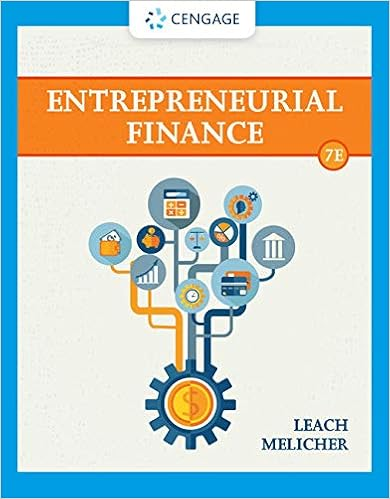# If the nominal interest rate on a bond is 59 and the

• Test Prep
• 20
• 100% (16) 16 out of 16 people found this document helpful

This preview shows page 2 - 6 out of 20 pages.

##### We have textbook solutions for you!
The document you are viewing contains questions related to this textbook.The document you are viewing contains questions related to this textbook.
Chapter 9 / Exercise 1
Entrepreneurial Finance
Leach/MelicherExpert Verified
7.If the nominal interest rate on a bond is 5.9% and the inflation rate is 3%, what is the realinterest rate of the bond? a.2.9%
##### We have textbook solutions for you!
The document you are viewing contains questions related to this textbook.The document you are viewing contains questions related to this textbook.
Chapter 9 / Exercise 1
Entrepreneurial Finance
Leach/MelicherExpert Verified
b.5.9% c.8.9% d.Not enough information
8.The table below presents long run macroeconomic data for two hypothetical nations: Nation Nominal GDP Growth Inflation Nominal Interest Rate Unemployment Rate Population Growth A 4% 1% 2% 5% B 10% 9% 11% 2% Assume both nations start with per capita real GDP of \$1000. Fill in the blanks in the table below assuming the data above applies to every year considered. 1% 0.5% Nation Economic Growth Rate Years required for real per capita GDP to double Real per capita GDP 140 years later A 4-1-1=2% 70/2=35 years 100*24=16,000 B 10-9-0.5=0.5% 70/0.5=140 years 1,000*2=2,000 9.A country has the production function Y= F(K,L). a.Draw an appropriate graph of capital and output to demonstrate this production function. Answer:
b.What happens to your output if you increase the amount of capital?
c.After some time, the production function changes to: Y=10.3 F(K,L). What is 10.3 in this situation (what does it represent generally)?
d.Which Solow Growth Model includes this variable?
e.Draw what the introduction of this variable does to the Solow graph in part (a)
10.Calculate the real per capita GDP growth rate for 2011 and 2012 (you may use a calculator, round to the nearest hundredth)
Year Nominal GDP (billions of \$) Inflation rate Population (millions) 2010 14,061 2% 2011 14,660 3% 2012 14,992 1% 112 114 117
•••